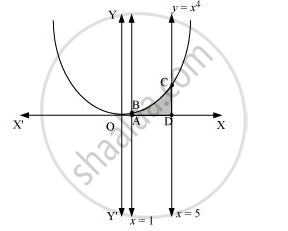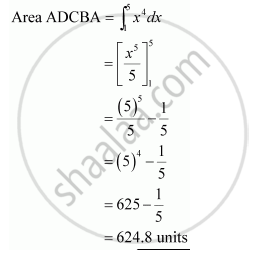Share
Notifications

View all notifications
Books Shortlist
Your shortlist is empty

# Find the Area Under the Given Curves and Given Lines: Y = X4, X = 1, X = 5 And X –Axis - CBSE (Commerce) Class 12 - Mathematics

Login
Create free account

Forgot password?

#### Question

Find the area under the given curves and given lines:

y = x4x = 1, x = 5 and x –axis

#### Solution

The required area is represented by the shaded area ADCBA asIs there an error in this question or solution?

#### APPEARS IN

NCERT Solution for Mathematics Textbook for Class 12 (2018 to Current)
Chapter 8: Application of Integrals
Q: 1.2 | Page no. 375
Solution Find the Area Under the Given Curves and Given Lines: Y = X4, X = 1, X = 5 And X –Axis Concept: Area Under Simple Curves.
S